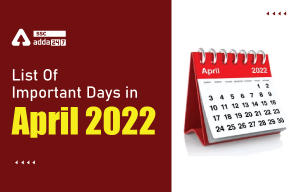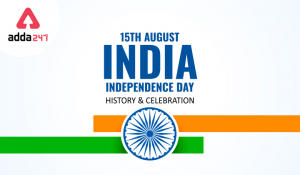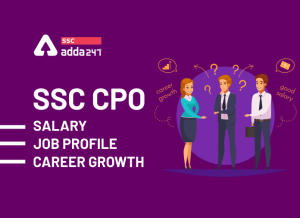Latest SSC jobs   »   How to Score 40+ Marks in...

# How to Score 40+ Marks in Quant Section of SSC CGL Tier-1 Exam

SSC CGL is near your doorstep all the appearing candidates must be knowing how important it is to score highly in tier 1 in order to move up in the pecking orders of the selection list. Scoring good marks in Tier-1 also provides an edge over other students during the tier-2 exams. Quantitative aptitude is a very important and scoring section and therefore it is a must to score good marks in the quants section. Candidates who have applied for the SSC CGL must be needing proper tips and guidance on how to score highly in the quants section in SSC CGL Tier-1. Keeping that in mind SSCAdda is providing some valuable tips for the same.
SSC CGL Tier-1 Exam Dates-> April 2022

Staff Selection Commission is conducting SSC CGL  2021-22 Tier 1 examination in April 2022. The SSC CGL Tier 1 all-shifts Quant questions will give you an insight into the topic carrying maximum marks. Candidates appearing in the upcoming shifts can go through the questions and prepare themselves for the shifts.

#### Quantitative Aptitude Questions Asked In SSC CGL Tier 1 Examination 13th August 2021:

1. cot 25 cot 35 cot 45 cot 55 cot 65=?
2. If x+y=4 , 1/x+1/y = 16/15 find x^3+y^3 = ?
3. If x+1/x=4 then find x^5 + 1/ x^5 =?
4. 676xy exactly divided by 3,7,11. Then 3x-5y=?
5. If side of a rhombus is 13 , one diagonal is 24. find the area of the rhombus?
6. 8(x+y)^3 – 27 (x-y) ^3 = (5y-x) ( Ax^2 + By^2 + Cxy). Find A+B-C
7. cosec a / (cosec a + 1) + cosec a/ (cosec a -1) – tan^2a =?
8. If 15 articles were purchased in rs.140, then 10 articles were sold in rs.60, and 12 articles were sold in Rs. 132. Calculate profit & loss.
9. cosec a / (cosec a + 1) + cosec a/ (cosec a -1) – tan^2a =?
10. if 2 cos^2a = 3 sin a then sec^2 a – tan ^2 a + cos ^2 a=?
11. If an article is sold at 176 after 12 % discount. If no discount is given he earns a profit of 25 %.then find CP?
12. x+1/x =5 , then find (x^6-5x^3+1)/(x^6+7x^3-1)
13. x-y=11,1/x-1/y = 11/24, then x^3 – y^3=?

Click Here For More Questions Asked in SSC CGL Tier 1 Examination: 13th August | All Shifts

Exam Date SSC CGL Tier 1: Shift-1
[10:00-11:00 am]
SSC CGL Tier 1: Shift-2
[1:00-2:00 pm]
SSC CGL Tier 1: Shift-3
[4:00-5:00 pm]

## SSC CGL Tier 1 Quantitative Aptitude questions asked in the previous years:-

For your convenience, we are providing the exact number of questions asked from respective topics in the Previous Year SSC CGL Tier 1 Exam in the Quant section. The number of questions asked from various topics remained almost the same, the only difference was in the difficulty level of the questions. Check out the table given below

S.No. Topics No. Of Questions asked in
SSC CGL 2016
SSC CGL 2017
SSC CGL 2018-19
SSC CGL 2019-20
1 Ratio 1 1 1 1
2 Average 1 1 1
3 Number System 1 1 1 2
4 Simplification 1 1
5 Time & Work 1 1 1
6 Time, Speed & Distance 1 1 1 1
7 S.I. & C.I. 1 1 1 1
8 Profit & Loss/ Discount 2 2 2 2
9 Coordinate Geometry
10 Geometry 5 4 4 2
11 Mensuration 2 1 1 3
12 Trigonometry 2 3 3 3
12 Percentage 1 1 1 1
12 Algebra 4 4 3 3
13 DI 4 4 4 4
Total Questions 25 25 25 25

In order to score high marks, first, you need to look for the topics containing the most number of questions and then start working on it. Just remember, Accuracy and Time Management is the key to score in Quantitative Aptitude. Let’s take a look at the strategy that needs to be adopted to prepare topic wise in the Quant section:

1. Geometry/Mensuration

This is one of the most important topics considering SSC CGL Tier 1 because over the years SSC has increased the number of questions asked from this topic. The level of difficulty has also been on the rise.

#### Click here to check the complete salary structure with in-hand salary here

For Geometry, you need to remember all the important theorems, Pythagoras triplet, all the formulas of the area of triangle and circle. Above all draw figures correctly to get a quick answer. A thorough understanding of the basics is the key to score well in this section.

2. Algebra/Trigonometry/Height and Distance

Algebra includes basic formulas of cubes, squares, trigonometric ratios and identities. Questions can be repeated from this section for which you need to practice the previous year papers. 2-3 questions will be asked from Algebra. Make sure to solve these questions in minimum time. Try to solve a few questions from the options given if you don’t find any way to solve them. Learn all the trigonometric identities useful for the exam. Overall 5-6 questions can be asked from Algebra, Trigonometry & Height and Distance.

3. Ratio/Percentage/profit, Loss and Discount

Basic Concepts of Percentage and ratio are the foundation of the chapters like profit, loss, and Discount. For Quick solving, you must remember all fractional values up to 30 in terms of percentage. Profit, loss, and discount revolve around 4 terms and that is C.P, S.P, M.P, and Discount. Understand the concept of CP, SP, MP, and discount given on MP and the relation between them. Practice from the books as much as you can. It will help you to solve the questions quickly and accurately.

4. Time speed and distance/ Train/ Boats and Stream

Inverse relation and direct relation between time, speed, and distance can help you a lot. The concept of relative velocity will help you in solving problems of Trains, Boats, and Stream. All these topics are based on the same concept. Gun and Bullet problem, Train accident problem, Effect of increasing/decreasing speed on time, these problems are often asked in exams. Overall 3-4 questions can be asked from these topics.

5. Time and Work/pipes and Cistern

Time and work questions can be solved with the help of the LCM method which reduces a lot of time while solving the questions. The concept of alternate work, efficiency, and work and wages are important points to cover. Make sure to use your own tricks for solving these questions.

6. Data Interpretation

DI is considered an easier topic in SSC CGL as compared to others. Do not take any topic or subject lightly as the exam can be full of surprises and you need to be prepared beforehand for what may come.

The best you could do is start taking online mock exams twice a day and analyse your performance thoroughly. For the rest of the time, start solving previous years’ questions. As you practice more, you will be able to solve the questions with a much better pace and this will surely help you boost your morale.

#### Congratulations!General Awareness & Science Capsule PDFIncorrect details? Fill the form again here

General Awareness & Science Capsule PDF

Thank You, Your details have been submitted we will get back to you.
•List of Important Days in April 2022, Na...
•Independence Day 2022, History & Cel...
•SSC CPO Salary: Job Profile, Allowances,...
•Happy Raksha Bandhan!!
•India's Biggest Govt Exams Book Fair, Fl...
•Merchant Navy Salary in India, Job Profi...